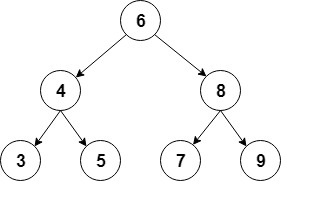java程序员当前位置：主页 > 高端课程 > java程序员 >

## 二叉树``````class Node {
int value;//本节点的值
Node left;//左边的子节点
Node right;//右边的子节点

Node(int value) {
this.value = value;
right = null;
left = null;
}
}``````

``````public class BinaryTree {

Node root;

// ...
}``````

## 让我们一起来实现下

### 插入元素

• 如果新节点的值低于当前节点的值，我们转到左子节点
• 如果新节点的值大于当前节点的值，我们将转到右子节点
• 节点当前为null时，我们已到达叶节点，我们可以在该位置插入新节点

``````private Node addRecursive(Node current, int value) {
if (current == null) {
return new Node(value);
}

if (value < current.value) {
} else if (value > current.value) {
} else {
return current;
}

return current;
}``````

``````public void add(int value) {
}``````

``````private BinaryTree createBinaryTree() {
BinaryTree bt = new BinaryTree();

return bt;
}``````

### 查找元素

``````private boolean containsNodeRecursive(Node current, int value) {
if (current == null) {
return false;
}
if (value == current.value) {
return true;
}
return value < current.value
? containsNodeRecursive(current.left, value)
: containsNodeRecursive(current.right, value);
}``````

``````public boolean containsNode(int value) {
return containsNodeRecursive(root, value);
}``````

``````@Test
BinaryTree bt = createBinaryTree();

assertTrue(bt.containsNode(6));
assertTrue(bt.containsNode(4));

assertFalse(bt.containsNode(1));
}``````

### 删除元素

``````private Node deleteRecursive(Node current, int value) {
if (current == null) {
return null;
}

if (value == current.value) {
// Node to delete found
// ... code to delete the node will go here
}
if (value < current.value) {
current.left = deleteRecursive(current.left, value);
return current;
}
current.right = deleteRecursive(current.right, value);
return current;
}``````

• 节点没有子节点 -这是最简单的情况; 我们只需要在其父节点中用 null 替换此节点
• 节点只有一个子节点 -在父节点中，我们用它唯一的子节点替换该节点。
• 节点有两个子节点 - 这是最复杂的情??况，因为它需要树重组

``````if (current.left == null && current.right == null) {
return null;
}``````

``````if (current.right == null) {
return current.left;
}

if (current.left == null) {
return current.right;
}``````

``````private int findSmallestValue(Node root) {
return root.left == null ? root.value : findSmallestValue(root.left);
}``````

``````int smallestValue = findSmallestValue(current.right);
current.value = smallestValue;
current.right = deleteRecursive(current.right, smallestValue);
return current;``````

``````public void delete(int value) {
root = deleteRecursive(root, value);
}``````

``````@Test
public void givenABinaryTree() {
BinaryTree bt = createBinaryTree();

assertTrue(bt.containsNode(9));
bt.delete(9);
assertFalse(bt.containsNode(9));
}``````

## 转换树

### 深度优先搜索

in-order：首先访问左子树，然后访问根节点，最后访问右子树：

``````public void traverseInOrder(Node node) {
if (node != null) {
traverseInOrder(node.left);
System.out.print(" " + node.value);
traverseInOrder(node.right);
}
}``````

``3 4 5 6 7 8 9``

pre-order：首先访问根节点，然后是左子树，最后是右子树：

``````public void traversePreOrder(Node node) {
if (node != null) {
System.out.print(" " + node.value);
traversePreOrder(node.left);
traversePreOrder(node.right);
}
}``````

``6 4 3 5 8 7 9``

post-order：访问左子树，右子树，最后访问根节点：

``````public void traversePostOrder(Node node) {
if (node != null) {
traversePostOrder(node.left);
traversePostOrder(node.right);
System.out.print(" " + node.value);
}
}``````

``3 5 4 7 9 8 6``

### 广度优先搜索

``````public void traverseLevelOrder() {
if (root == null) {
return;
}

while (!nodes.isEmpty()) {

Node node = nodes.remove();

System.out.print(" " + node.value);

if (node.left != null) {
}

if (node.right!= null) {
}
}
}``````

``6 4 8 3 5 7 9``

## 最后

“不积跬步，无以至千里”，希望未来的你能成为：有梦为马 随处可栖！加油，少年！

• 全球最大晶圆代工半导体制造厂，台积电斥资订购艾斯摩尔机器设备
• 英特尔依然是那个英特尔，且看英特尔的城防体系
• 支持双 DRAM 内存接口，慧荣企业级 SSD 主控方案披露
• 在全球被反垄断罚款，冤！高通到底哪里得罪了欧盟？
• 强强联合！万业、微电子所和芯鑫共同打造全新半导体设备
• 复旦大学校长称：对于集成电路产业发展，大学应该主动担当
• 技术再升级！无锡中科芯攻克晶圆级再布线及晶圆级凸点制备关键技
• 聚力！万业企业设立集成电路装备集团，提供自主可控设备
• 德州仪器C2000微控制器增强连通性和控制性
• 英特尔打出降价策略，以免被竞争对手 AMD 打败？
• 贸易摩擦的闹剧没有赢家，苹果有勇气离开中国吗？
• 图像信号与视觉处理器的发展趋势
• 真干快消品定位方案班（第二期）火热开班
• Java集合 ArrayList原理及使用
• TDD（测试驱动开发）死了吗？
• JAVA基础之XML相关
• javaweb项目搭建ehcache缓存系统
• 每日一码——字符串统计
• 一篇文章帮你彻底搞清楚“I/O多路复用”和“异步I/O”的前世今生
• 九：模板方法模式
• 十二：命令模式（人员解耦和）
• Java 转PPT为图片、PDF、SVG、XPS、ODP以及PPT和PPTX互转
• SpringCloud学习（SPRINGCLOUD微服务实战）一
• 记一次微信网页授权后获取用户信息并重定向
• 速途新营销五点实战洞察解码“品效合一”
• 十一：外观模式详解（Service，action与dao）
• 手把手教你学会 基于JWT的单点登录
• mysql锁机制总结，以及优化建议
• 解决多个版本jar包冲突【jar内包名重命名】
• 中国首张5G终端电信设备进网许可证 华为Mate 20 X 5G版入网
• RPC之Thrift
• 高级Java工程师必备 ----
• 天猫618期间实物支付GMV增长38.5%
• 换季了，老板你的库存处理好了吗？
• 从“618”大数据看中国消费新活力
• 小米生态链：贵在格局感与收放度
• CODING 2.0 企业级持续交付解决方案
• 老铁奇趴“新京济” 快手*京东618战报出炉
• 中小企业新媒体运营基本技能
• 上汽大通房车再度携手LINE FRIENDS 魔都巡游顺利开启
• 华为高端手机国内市场份额超苹果夺得榜首
• 中国智能制造分析报告
• iPlus艾加营销助力腾讯广告牵手吴晓波 推进商业IP变现
• 2019世界新能源汽车大会7月1日将在海南举行
• 区域酒企如何转型突围
• 时时彩论坛
• 五星体育斯诺克
• 北单比分直播
• 河北11选5走势图
• 福建体彩36选7开奖结果
• 九龙图库下载